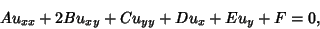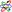## Parabolic Partial Differential Equation

A Partial Differential Equation of second-order, i.e., one of the form(1)

is called parabolic if the Matrix(2)

satisfies det. The Heat Conduction Equation and other diffusion equationsare examples. Initial-boundary conditions are used to give(3)(4)

where(5)

holds in.

See also Elliptic Partial Differential Equation, Hyperbolic Partial Differential Equation, Partial Differential Equation